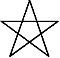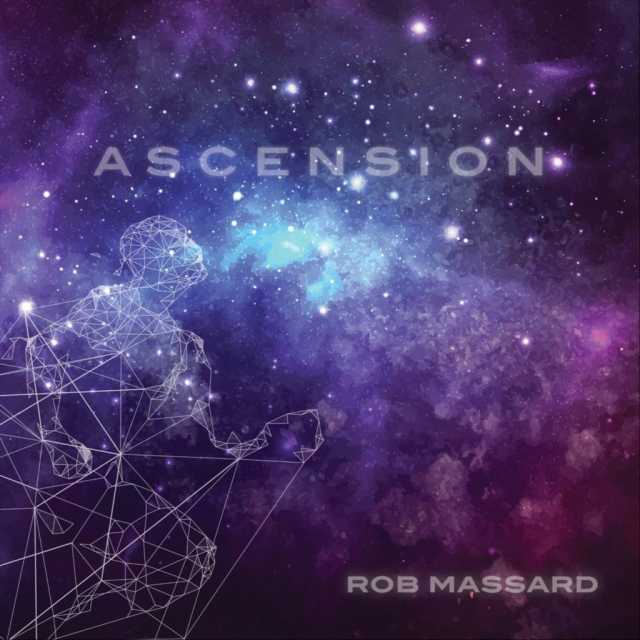Art, History, Music, Politics, and Spirituality For The Modern Alchemist – circulation in over 129 countries

# Sign of Sheba Year 18,004: Begins June 17th 2016

There are eight months in the Calendar of Mu, sometimes referred to as the Nyarzirian Calendar. Each month consists of forty-five days, and represents a system of the eight hexagrams moving through the five elements. The Ninzuwu Priest/Priestess invokes the mudra/mantra formulae associated with each hexagram that rules a particular day after invoking the Armor of Amaterasu Ohkami. The chart below illustrates the daily Vasuh letters invoked and the ruling “deity” for the said hexagram:
1. June 17th, 2016 = Tasuta = 9th Hexagram = Hmu-Bnhu
2. June 18th 2016 = Kukunochi = 20th Hexagram = Hmu-Tuu
3. June 19th 2016 = Ieh = 37th Hexagram = Zhee-Zhee-Lewhu
4. June 20th 2016 = Gzk = 42nd Hexagram = Zhee-Tuu-Hmu-Nzu-Aum
5. June 21st 2016 = Jhn = 53rd Hexagram = Zhee-Bnhu
6. June 22nd 2016 = Sheba = 57th Hexagram = Hmu-Shki-Bnhu-Bnhu
7. June 23rd 2016 = Lzwa = 59th Hexagram = Aum-Shki
8. June 24th 2016 = Kuhvi = 61st Hexagram = Phe-Phe
9. June 25th 2016= Entering Earth Element
10. June 26th 2016 = Tasuta = 9th Hexagram = Hmu-Bnhu
11. June 27th 2016 = Kukunochi = 20th Hexagram = Hmu-Tuu
12. June 28th 2016 = Ieh = 37th Hexagram = Zhee-Zhee-Lewhu
13. June 29th 2016 = Gzk = 42nd Hexagram = Zhee-Tuu-Hmu-Nzu-Aum
14. June 30th 2016 = Jhn = 53rd Hexagram = Zhee-Bnhu
15. July 1st  2016 = Sheba = 57th Hexagram = Hmu-Shki-Bnhu-Bnhu
16. July 2nd 2016 = Lzwa = 59th Hexagram = Aum-Shki
17. July 3rd 2016 = Kuhvi = 61st Hexagram = Phe-Phe
18. July 4th  2016=  Entering Water Element
19. July 5th  2016 = Tasuta = 9th Hexagram = Hmu-Bnhu
20. July 6th 2016 = Kukunochi = 20th Hexagram = Hmu-Tuu
21. July 7th 2016 = Ieh = 37th Hexagram = Zhee-Zhee-Lewhu
22. July 8th 2016 = Gzk = 42nd Hexagram = Zhee-Tuu-Hmu-Nzu-Aum
23. July 9th 2016 = Jhn = 53rd Hexagram = Zhee-Bnhu
24. July 10th 2016 = Sheba = 57th Hexagram = Hmu-Shki-Bnhu-Bnhu
25. July 11th 2016 = Lzwa = 59th Hexagram = Aum-Shki
26. July 12th 2016 = Kuhvi = 61st Hexagram = Phe-Phe
27. July 13th 2016=  Entering Fire Element
28. July 14th 2016 = Tasuta = 9th Hexagram = Hmu-Bnhu
29. July 15th 2016 = Kukunochi = 20th Hexagram = Hmu-Tuu
30. July 16th 2016 = Ieh = 37th Hexagram = Zhee-Zhee-Lewhu
31. July 17th 2016 = Gzk = 42nd Hexagram = Zhee-Tuu-Hmu-Nzu-Aum
32. July 18th 2016 = Jhn = 53rd Hexagram = Zhee-Bnhu
33. July 19th 2016 = Sheba = 57th Hexagram = Hmu-Shki-Bnhu-Bnhu
34. July 20th 2016 = Lzwa = 59th Hexagram = Aum-Shki
35. July 21st 2016 = Kuhvi = 61st Hexagram = Phe-Phe
36. July 22nd 2016=  Entering Air Element
37. July 23rd 2016 = Tasuta = 9th Hexagram = Hmu-Bnhu
38. July 24th 2016 = Kukunochi = 20th Hexagram = Hmu-Tuu
39. July 25th 2016 = Ieh = 37th Hexagram = Zhee-Zhee-Lewhu
40. July 26th 2016 = Gzk = 42nd Hexagram = Zhee-Tuu-Hmu-Nzu-Aum
41. July 27th 2016 = Jhn = 53rd Hexagram = Zhee-Bnhu
42. July 28th 2016 = Sheba = 57th Hexagram = Hmu-Shki-Bnhu-Bnhu
43. July 29th 2016 = Lzwa = 59th Hexagram = Aum-Shki
44. July 30th 2016 = Kuhvi = 61st Hexagram = Phe-Phe
45. July 31st 2016=  Entering Void Element

#### You may have missed#### October by Mae Simpson#### Night Owl by Adrian John Szozda